# Beginning Algebra Review Exam 2## Material covered: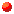Like Terms; the Distributive Law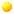Solving Equations; Applied Problems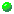Graphing Equations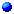Slope of a Line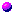Writing Equations of Lines

```I.  Simplify:

A.  8 - 3(12x - 4) - 2x
B.  (25x + 9) - (-55x + 23)

II.  Solve for x:

A.  5(3x - 2) = 35
B.  16x - 3(15x + 8) - 6 = 3(5x - 4) - 4(8x - 5)

III.  Write each of the following equations in explicit form (i.e. solve equation for y)
and find three solutions to the equation and then graph and then graph.

A.  x + 2y = 1
B.  2x - y = 4
C.  y - 3x = 0

IV.  Graph each of the following lines.

A.  x = -2
B.  3x - 15 = 0
C.  y = 3
D.  2y + 4 = 0

V.  Complete the following statements
A.  To find the x-intercept of a line ______________________________________________
B.  To find the y-intercept of a line ______________________________________________
C.  Find the x-intercept and the y-intercept of each of the following lines and then graph.

1.  7y - 4x = 28		2.  3x - 15 = 15y

VI.  Write each of the following equations in slope-intercept form, i.e. of the form y = mx + b.
Determine the slope and y-intercept of each of the following and then graph.

A.  2y + x = 6
B.  4y - 12 = 0
C.  6x + 3y =9

VII.  Given the following lines.  Determine the slope of each line, the slope of any line parallel
to the given line, and the slope of any line perpendicular to the line.

A.  3x - y = 1
B.  2y + 3x = 6

VIII.  Find the equation of each of the following lines.  Express the equation of the form
Ax + By + C = 0 where A, B, and C are integers.

A.  The line through (-2,3) with slope 5.
B.  The line passing through (-1,2) and (3,10).
C.  The line passing through (4,-1) which is parallel to the line y = 2x - 5.
D.  The line passing through (4,-1) perpendicular to the line y = 2x - 5

IX.  State the following formulas.

A.  Slope Formula
B.  Point-Slope Formula
C.  Slope-Intercept Formula

X.  Given line one with slope m and line two with slope n
.
A.  Line one is parallel to line two if and only if __________________________
B.  Line one is perpendicular to line two if and only if _____________________

XI.  Stated Problems:

A.  Problem 33 page 153
B.  Problem 23 page 152
C.  Problem 7 page 165
D.  Problem 19 page 166
E.  Problem 31 page 167```